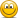# CourseErrors Found in 2021/2022 Study Materials P2.T8. Liquidity and Treasury Risk

#### Nicole Seaman

##### Director of FRM Operations
Staff member
Subscriber
Please use this new thread to let David and I know about any errors, missing/broken links, etc. that you find in the 2021/2022 materials that are published in the study planner under P2.T8. Liquidity and Treasury Risk. This will keep our forum much more organized. We appreciate your cooperation!PLEASE NOTE: Our Practice Question sets already have links to their specific forum threads where you can post about any errors that you find. This thread is for any other materials (notes, spreadsheets, videos, etc.) where you might find errors.

Information needed for us to correct errors:

• Page number
• Error

Last edited:

#### sohinichowdhury

##### Member
Reading: Ang, Illiquid Assets (Chapter 13).
Page 16
In the third equation, the coefficient on the lagged observed return is missing a theta in the numerator.

#### sohinichowdhury

##### Member
I believe this is an error (can someone please confirm?)

Reading: Rose & Hudgins Chapter 7
Page: Top lines of page 26
Error: The 4 words with a strike out in these lines should read the opposite.

Essentially, if the leverage duration gap is positive, then a parallel change in all interest rates will cause liabilities to change by less than assets. Consequently, if interest rates fall, liabilities will increase more than assets and the firm’s net worth will decline. On the flip side, if interest rates rise, liability values will fall faster than asset values and the net worth of the firm will increase.

#### Hamam

##### Active Member
Hi David,
Just wanted to let you know that the study notes (Castagna Ch.6) need to be amended slightly, the chart on the last page ( I think this table were from the books) says that the TSECF & the TSECCF is changed for the reverse repo agreement, but in this transaction ownership is with the counter party so actually for the bank these term structures don't change.
Thanks

Subscriber

#### bollengc

##### Member
Subscriber
hello,

in T8-R44-P2-Hull-RMFI-Ch24-v3.pdf, page 3, s notation is used for both the spread and the proportional bid-offer spread.

as well, there might be some typo in the sentence highlighted in yellow. I guess what was meant was "the proportional bid-offer spread is simply this equation divided by the mid-market price."As well, as mentioned in https://forum.bionicturtle.com/thre...and-treasury-risk-old-thread.23017/post-85229, the numerical example on page 4 and 5 is not good.
Mid market values on page 4 are ok but the rest of the computation does not make sense.If you were to apply the computation of the last line, you would even get $150,000,000 and not$105,011,875.

but the good value should be: (100.5-100)/100.25*(100 mil/2*100.25) + (20.1-20)/20.05*(200mil/2*20.05)

Last edited:

#### AChau2828

##### New Member
Subscriber
Liquidity practice question --

20.2.2. A position with a value of $2.0 million consists of 50,000 shares of stock. The stock is quoted bid$39.00, offer $41.00. The bid-offer spread has a volatility of 2.0%. The stock's daily volatility is 2.00% or 200 basis points. For purposes of value at risk (VaR), assume the stock's arithmetic returns are normally distributed (aka, normal VaR) and the expected daily return rounds to zero (under these assumptions absolute VaR is identical to relative VaR). We want to measure the cost of liquidity under stressed market conditions; aka, we will assume a worst- case volatile spread rather than a static spread. Which is NEAREST to the position's one-day 95.0% confident liquidity-adjusted value at risk (LVaR)? (Please assume a rounded normal deviate of 1.65).$76,300
$149,000$318,000
$755,000 . 1. Solution - B. True:$149,000
•  The one-day 95.0% VaR is given by -0 + 2.00% * 1.65 * 2.0 million = $66,000. •  The volatile-spread liquidity cost (LC) in percentage terms is given by 0.5 * (5.0% + 2.0% * 1.65) = 4.150%, such that the liquidity cost equals$2.0 million * 4.150% = $83,000. •  Therefore, the one-day 95.0% LVaR equals$66,000 + $83,000 =$149,000 (using an exact normal deviate, the answer is $148,691). Question - how was the 5% mean spread % was calculated or was it assumed? #### bollengc ##### Member Subscriber Liquidity practice question -- 20.2.2. A position with a value of$2.0 million consists of 50,000 shares of stock. The stock is quoted bid $39.00, offer$41.00. The bid-offer spread has a volatility of 2.0%. The stock's daily volatility is 2.00% or 200 basis points. For purposes of value at risk (VaR), assume the stock's arithmetic returns are normally distributed (aka, normal VaR) and the expected daily return rounds to zero (under these assumptions absolute VaR is identical to relative VaR). We want to measure the cost of liquidity under stressed market conditions; aka, we will assume a worst- case volatile spread rather than a static spread.
Which is NEAREST to the position's one-day 95.0% confident liquidity-adjusted value at risk (LVaR)? (Please assume a rounded normal deviate of 1.65).
$76,300$149,000
$318,000$755,000
.
1. Solution - B. True: $149,000 •  The one-day 95.0% VaR is given by -0 + 2.00% * 1.65 * 2.0 million =$66,000.
•  The volatile-spread liquidity cost (LC) in percentage terms is given by 0.5 * (5.0% + 2.0% * 1.65) = 4.150%, such that the liquidity cost equals $2.0 million * 4.150% =$83,000.
•  Therefore, the one-day 95.0% LVaR equals $66,000 +$83,000 = $149,000 (using an exact normal deviate, the answer is$148,691).
Question - how was the 5% mean spread % was calculated or was it assumed?

#### bollengc

##### Member
Subscriber
hello David (@David Harper CFA FRM )

in T8-R58-P2-Borio-v3, bottom of page 4 + part up of page 5, I really have difficulty understanding the different steps

starting point:Suppose for simplicity that the real interest rate in the foreign currency is sufficiently small to be
negligible.how do we end up to the first part of the equation, and then to the part but the bid and ask values?thanks,
Camille